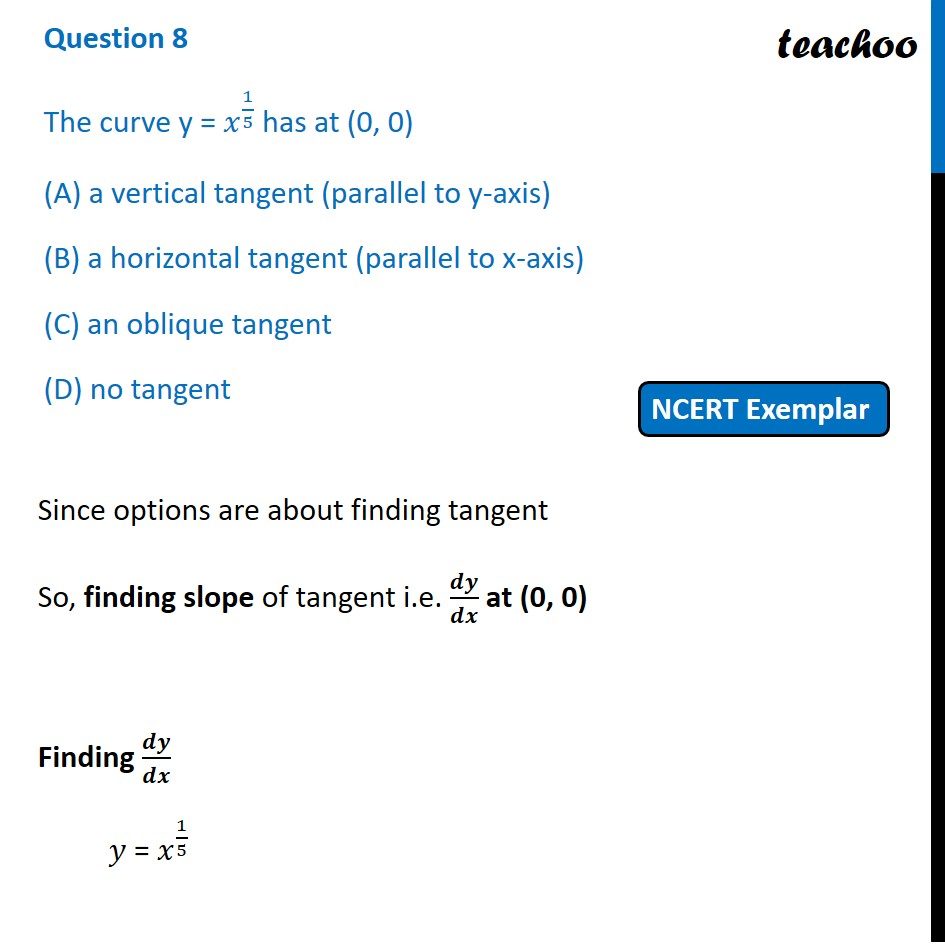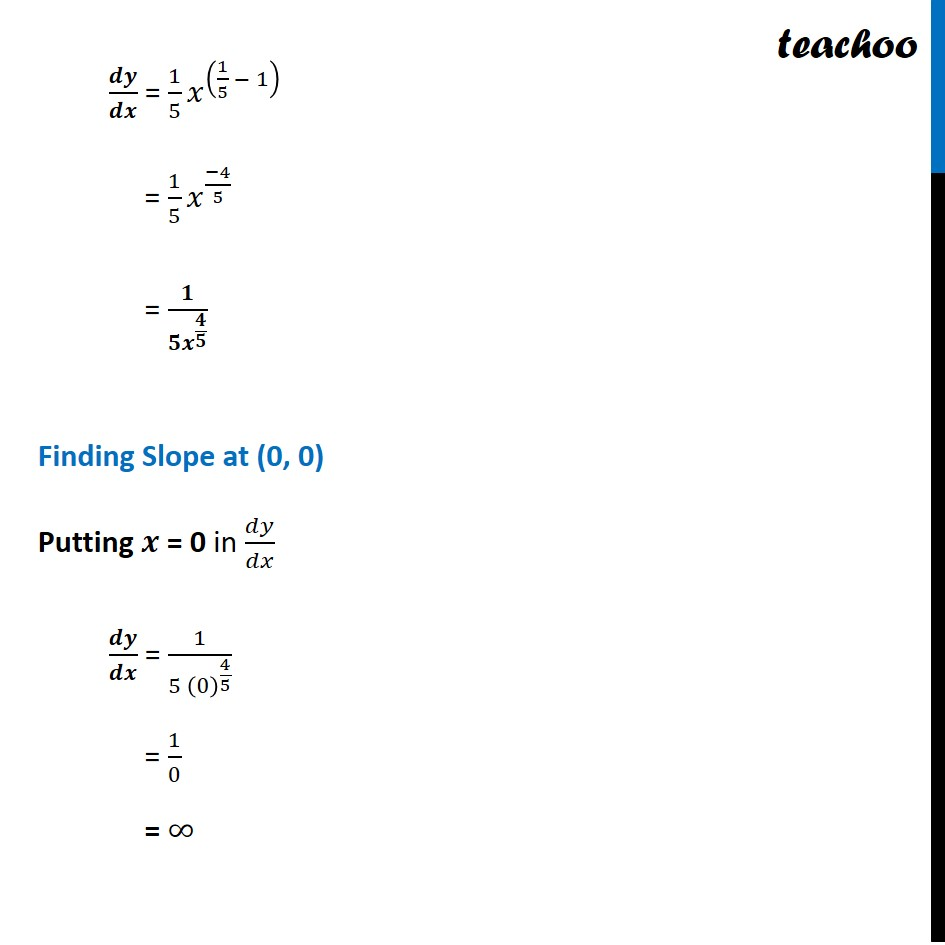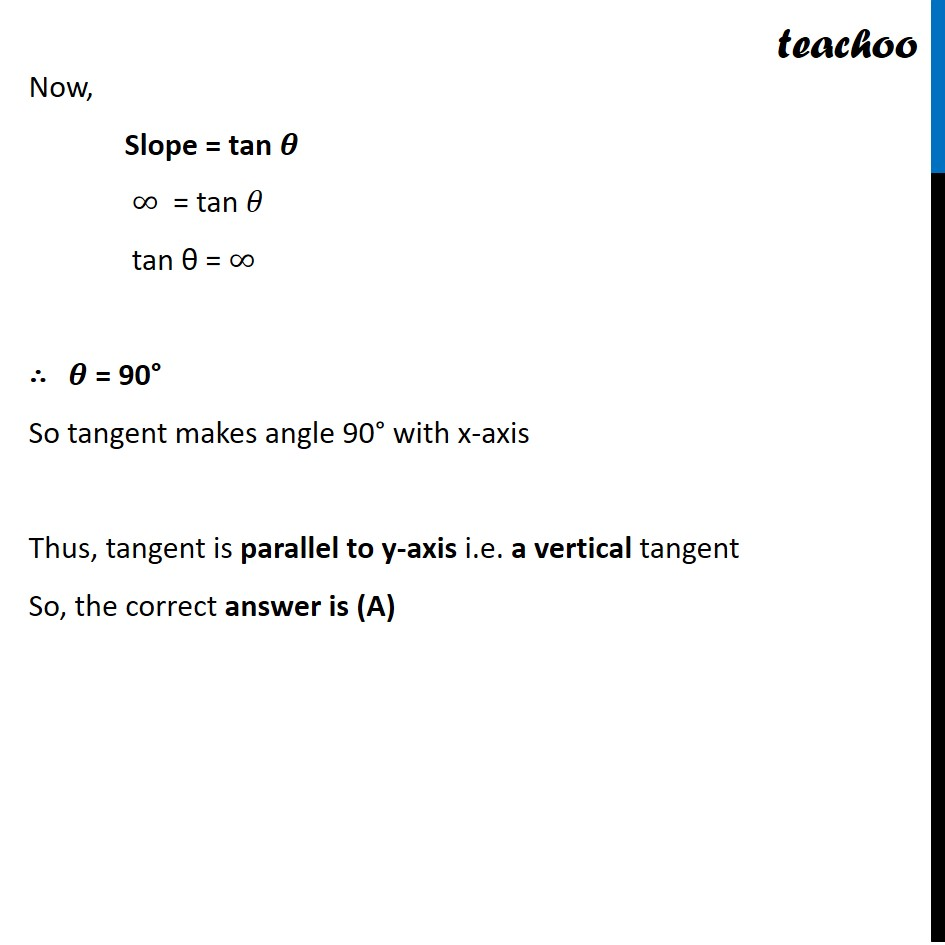NCERT Exemplar - MCQs

Chapter 6 Class 12 Application of Derivatives
Serial order wise

## (D) no tangentLearn in your speed, with individual attention - Teachoo Maths 1-on-1 Class

### Transcript

Question 6 The curve y = 𝑥^(1/5) has at (0, 0) (A) a vertical tangent (parallel to y-axis) (B) a horizontal tangent (parallel to x-axis) (C) an oblique tangent (D) no tangent Since options are about finding tangent So, finding slope of tangent i.e. 𝒅𝒚/𝒅𝒙 at (0, 0) Finding 𝒅𝒚/𝒅𝒙 𝑦 = 𝑥^(1/5) 𝒅𝒚/𝒅𝒙 = 1/5 𝑥^((1/5 − 1) ) = 1/5 𝑥^((−4)/5) = 𝟏/(𝟓𝒙^(𝟒/𝟓) ) Finding Slope at (0, 0) Putting 𝒙 = 0 in 𝑑𝑦/𝑑𝑥 𝒅𝒚/𝒅𝒙 = 1/(5 (0)^(4/5) ) = 1/0 = ∞ Now, Slope = tan 𝜽 ∞ = tan 𝜃 tan θ = ∞ ∴ 𝜽 = 90° So tangent makes angle 90° with x-axis Thus, tangent is parallel to y-axis i.e. a vertical tangent So, the correct answer is (A)